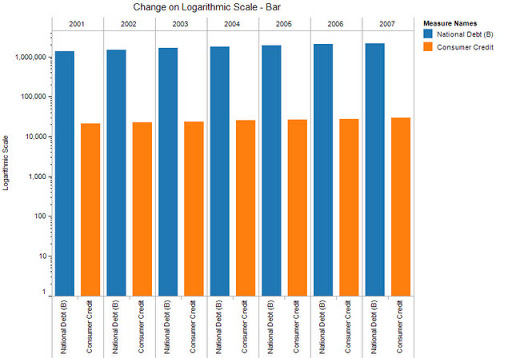Data Viz Done Right

# Should you use a log scale vs. zero-based scale for comparisons?

I finished re-reading Show Me the Numbers by Stephen Few last week and in Chapter 10 there is a discussion about Scale Lines, a subsection of which is devoted to logarithmic scales. Stephen made one particular recommendation that caught my eye (p. 199):
"Whenever you want to compare differences in values as a ratio or percentage, logarithmic scales will do the job nicely. They are especially useful in time-series relationships if you wish to compare ratios of change across time."

On p. 200, Few goes on to say:
"When you use lines to encode time-series along a logarithmic scale, angles that are the same tell you that the rate of change is the same."

Personally, I try, whenever possible, to avoid using a logarithmic scale. They tend to be very difficult to interpret and, for me, it's much easier to understand a graph that shows % change on a zero-based axis. Let's look at an example of both starting with a bar chart.On this bar chart, it's challenging, but you could make the assumption that both bars are changing at the same rate. This same chart is much easier to read as a line chart like the following:On this line chart, it is much easier to see how both line have roughly the same angle of ascent leading you to believe that they have approximately equal rates of change.

However, if you look at the same line chart, but with a zero-based axis, you get a much different message:You can now much more clearly see that the percentage change year to year for the two measures is very different. (NOTE: I have hidden 2001 from this chart because it is the reference point from which the measures begin.) In fact, the logarithmic scale would lead you to believe that there is a positive percent change, however, it is clear from this zero-based line chart that it's quite to opposite.

In the end, I urge caution when using logarithmic scale and my suggestion would be to use a zero-based scale to represent percentage change whenever you can.